# Decomposition

Mathematics too has profited from the idea that sometimes things of interest might have a structure which allowed them to be decomposed into simpler parts...

Joe Malkevitch
York College (CUNY)

### Introduction

One way to get insights into something one is trying to understand better is to break the thing down into its component parts, something simpler. Physicists and chemists found this approach very productive—to understand water or salt it was realized that common table salt was sodium chloride, a compound made of two elements, sodium and chlorine and that water was created from hydrogen and oxygen. Eventually, many elements (not the Elements of Euclid!) were discovered. The patterns noticed in these building block elements lead to the theoretical construct called the periodic table, which showed that various elements seemed to be related to each other. The table suggested that there might be elements which existed but had not been noticed; the "holes" in the table were filled when these elements were discovered, sometimes because missing entries were sought out. The table also suggested "trans-uranium" elements, which did not seem to exist in the physical world but could be created, and were created, in the laboratory. These new elements were in part created because the periodic table suggested approaches as to how to manufacture them. The work done related to understanding the structure of the periodic table suggested and lead to the idea that elements were also made up of even smaller pieces. This progression of insight lead to the idea of atoms, and that atoms too might have structure lead to the idea of subatomic particles. But some of these "fundamental" particles could be decomposed into smaller "parts." We now have a zoo of quarks and other "pieces" to help us understand the complexities of the matter we see in the world around us.Crystals of gallium, an element whose existence was predicted using the periodic table
Photo by Wikipedia user Foobar, CC BY-SA 3.0

### Prime patterns

Mathematics too has profited from the idea that sometimes things of interest might have a structure which allowed them to be decomposed into simpler parts. A good example is the number 111111111. It is an interesting pattern already, because all of its digits are 1's when written in base 10. We could compare 111111111 with the number that it represents when it is interpreted in base 2 (binary)—here it represents 511. But it might be interesting to study any relation between numbers with all 1's as digits and compare them to numbers in other bases, not only base 2! Mathematics grows when someone, perhaps a computer, identifies a pattern which can be shown to hold in general, rather than for the specific example that inspired the investigation. A number of the form 1111....1 is called a repunit. Can we find interesting patterns involving repunits?

One approach to decomposing a number (here strings of digits are to be interpreted as being written in base 10) is to see if a number can be written as the product of two other numbers different from 1 and itself. For example, 17 can only be written as the product of the two numbers 17 and 1. On the other hand, 16 can be written as something simpler, as $2 \times 8$ but there are "simpler" ways to write 16, as $4 \times 4$, and since 4 can be decomposed as $2 \times 2$ we realize that 16 can be written as $2 \times 2 \times 2 \times 2$. Seeing this pattern, mathematicians are trained to ask questions, such as whether numbers which are all the products of the same number which cannot be broken down have any special interest. But we are getting ahead of ourselves here. What are the "atoms" of the multiplicative aspect of integers? These are the numbers called the primes, 2, 3, 5, 7, 11, ... Notice that 11 is also a repunit. This takes us back to the idea that there might be many numbers of the form 11111.......111111 that are prime! Are there infinitely many primes all of whose digits in their base 10 representation are one? Answer!! No one knows. But it has been conjectured that there might be infinitely many repunit primes. Note that numbers like 111, 111111, 111111111, ... where the number of digits is a multiple of 3, can't be prime.

Note 11 base 2 is 3, which is also prime. Similarly, 1111111111111111111 is prime, and 1111111111111111111 base 2 represents the number 524287, which is also prime. If a repunit is prime, must the decimal number it represents treated as a base 2 number be prime?

By looking for "parts" that were building blocks for the integers, mathematics has opened a rich array of questions and ideas many of which have spawned major new mathematical ideas both theoretical and applied. Having found the notion of prime number as a building block of the positive number system, there are natural and "unnatural" questions to ask:

1. Are there infinitely many different primes?
2. Is there a "simple" function (formula) which generates all of the primes, or if not all primes, only primes?

While the fact that there are infinitely many primes was already known by the time of Euclid, the irregularity of the primes continues to be a source of investigations to this day. Thus, the early discovered pattern that there seemed to be pairs of primes differing by two (e.g. 11 and 13, 41 and 43, 139 and 141), which lead to the "guess" that perhaps there are infinitely many numbers of the form $p$ and $p+2$ that are both primes (known as twin primes) is still unsolved today. While more and more powerful computers made possible finding larger and larger twin prime pairs, no one could find a proof of the fact that there might be infinitely many such pairs. There were attempts to approach this issue via a more general question. Were there always primes which were some fixed bound of numbers apart? Little progress was made on this problem until in 2013 a mathematician whose name was not widely known in the community showed that there was a large finite uniform bound on a fixed size gap which could appear infinitely many times. This work by Yitang Zhang set off a concerted search to improve his methods and alter them in a way to get better bounds for the size of this gap. While Zhang's breakthrough has been improved greatly, the current state of affairs is still far from proving that the twin-prime conjecture is true.Photo of Yitang Zhang
Courtesy of Wikipedia

Mathematical ideas are important as a playground in which to discover more mathematical ideas, thus enriching our understanding of mathematics as an academic subject and sometimes making connections between mathematics and other academic subjects. Today there are strong ties between mathematics and computer science, an academic subject that did not even exist when I was a public school student. Mathematics can be applied in ways that not long ago could not even be imagined, no less carried out. Who would have thought that the primes would help make possible communication that prevents snooping by others as well as protecting the security of digital transactions? From ancient times, codes and ciphers were used to make it possible to communicate, often in military situations, so that should a communication fall into enemy hands it would not assist them. (Codes involve the replacement of words or strings of words with some replacement symbol(s), while ciphers refer to replacing each letter in an alphabet with some other letter in order to disguise the meaning of the original text.) Human ingenuity has been remarkable in developing clever systems for carrying out encryption of text rapidly and has allowed the receiver to decrypt the message in a reasonable amount of time but would, at the very least, slow down an enemy who came into possession of the message. But the development of calculators and digital computers made it harder to protect encrypted messages, because many systems could be attacked by a combination of brute force (try all possible cases) together with using ideas about how the design of the code worked. There was also the development of statistical methods based on frequency of letters and/or words used in particular languages that were employed to break codes. You can find more about the interactions between mathematics, ciphers, and internet security in the April 2006 Feature Column!

Earlier we looked at "decomposing" numbers into their prime parts in a multiplication of numbers setting. Remarkably, a problem about decomposing numbers under addition has stymied mathematics for many years, despite the simplicity of stating the problem. The problem is named for Christian Goldbach (1690-1764).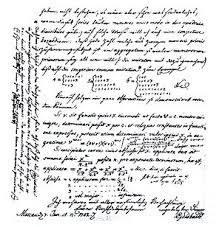Letter from Goldbach to Euler asking about what it is now known as Goldbach's Conjecture
Courtesy of Wikipedia

#### Goldbach's Conjecture (1742)

Given an even positive integer $n$, $n$ can be written as the sum of two primes.

For example, $10 = 3 + 7$ or also $5 + 5$, $20 = 3 + 17$, $30 = 11 + 19$. We allow the primes to be either the same or different in the decomposition. While computers have churned out larger and larger even numbers for which the conjecture is true, the problem is still open after hundreds of years.

What importance should one attach to answering a particular mathematical question? This is not an easy issue to address. Some mathematical questions seem to be "roadblocks" to getting insights into what seem to be important questions in one area of mathematics and in some cases answering a mathematical question seems to open doors on many mathematical issues. Another measure of importance might be in terms of aesthetic properties of a particular mathematical result. The aesthetic may be from the viewpoint that something seems surprising or unexpected or the aesthetic may be that a result seems to have "beauty"—a trait that whether one is talking about beautiful music, fabrics, poems etc. seems to differ greatly from one person to the next. It is hard to devise an objective yardstick for beauty. Another scale of importance is the "value" of a mathematical result to areas of knowledge outside of mathematics. Some results in mathematics have proved to be insightful in many academic disciplines like physics, chemistry, biology but other mathematics seems only to be relevant to mathematics itself. What seems remarkable is that over and over again mathematics that seemed only to have value within mathematics itself or to be only of "theoretical" importance, has found use outside of mathematics. Earlier I mentioned some applications of mathematical ideas to constructing ciphers to hide information. There are also codes designed to correct errors in binary strings and to compress binary strings. Cell phones and streaming video use these kinds of ideas: it would not be possible to have the technologies we now have without the mathematical ideas behind error correction and data compression.

### Partitions

The word decompose has some connotations in common with the word partition. Each of these words suggests breaking up something into pieces. Often common parlance guides the use of the technical vocabulary that we use in mathematics, but in mathematics one often tries to be very careful to be precise in what meaning one wants a word to have. Sometimes in popularizing mathematics this attempt to be precise is the enemy of the public's understanding the mathematics involved. Sometimes precise words are used to define a concept which are mathematically precise but obscure the big picture of what the collection of ideas/concepts that are being defined precisely are getting at. Here I try to use "mathematical terminology" to show the bigger picture of the ideas involved.

Given a positive integer $n$, we can write $n$ as a sum of positive integers in different ways. For example, $3 = 3$, $3 = 2+1$, and $3 = 1 + 1 + 1$. In counting the number of decompositions possible, I will not take order of the summands into account—thus, $1 +2$ and $2 +1$ will be considered the same decomposition. Each of these decompositions is considered to be a partition of 3. In listing the partitions of a particular number $n$, it is common to use a variant of set theory notation where the entries in set brackets below can be repeated. Sometimes the word multiset is used to generalize the idea of set, so that we can repeat the same element in a set. Thus we can write the partitions of three as $\{3\}$, $\{2,1\}$, $\{1,1,1\}$. A very natural question is to count how many different partitions there are of $n$ for a given positive integer. You can verify that there are 5 partitions of the number 4, and 11 partitions of the number 5. Although for very large values of $n$ the number of partitions of $n$ has been computed, there is no known formula which computes the number of partitions of $n$ for a given positive integer $n$. Sometimes the definition of partition insists that the parts making up the partition be listed in a particular order. It is usual to require the numbers in the partition not to increase as they are written out. I will use this notational convention here: The partitions of 4 are: $\{4\}$, $\{3,1\}$, $\{2, 2\}$, $\{2, 1, 1\}$, $\{1,1,1,1\}$. Sometimes in denoting partitions with this convention exponents are used to indicate runs of parts: $4$; $3,1$; $2^2$; $2, 1^2$; $1^4$. The notation for representing partitions varies a lot from one place to another. In some places for the partition of 4 consisting of $2 + 1 + 1$ one sees $\{2,1,1\}$, $2+1+1$, $211$ or $2 1^2$ and other variants as well! It may be worth noting before continuing on that we have looked at partitions of $n$ in terms of the sum of smaller positive integers but there is another variant that leads in a very different direction. This involves the partition of the set $\{1,2,3,\dots,n\}$ rather than the partition of the number $n$. In this framework the partition of a set $S$ consists of a set of non-empty subsets of the set $S$ whose union is $S$. (Remember that the union of two sets $U$ and $V$ lumps together the elements of $U$ and $V$ and throws away the duplicates.)

Example: Partition the set $\{1,2,3\}$:

Solution:

$$\{1,2,3\}, \{1,2\} \cup \{3\}, \{1, 3\} \cup \{2\}, \{2,3\} \cup \{1\}, \{1\} \cup \{2\} \cup \{3\}$$

While there are 3 partitions of the number 3 there are 5 partitions of the set $\{1,2,3\}$. The number of partitions of $\{1,2,3,\dots,n\}$ are counted by the Bell numbers, developed by Eric Temple Bell (1883-1960). While the "standard" name for these numbers now honors Bell, other scholars prior to Bell also studied what today are known as the Bell numbers, including the Indian mathematician Srinivasa Ramanujan (1887-1920).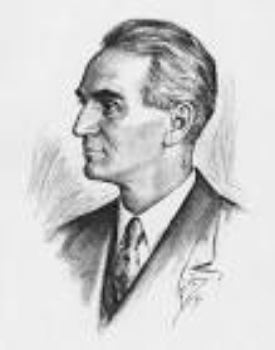A sketch of Eric Temple Bell
Courtesy of Wikipedia

Partitions have proved to be a particularly intriguing playground for studying patterns related to numbers and have been used to frame new questions related to other parts of mathematics. When considering a partition of a particular number $n$, one can think about different properties of the entries in one of the partitions:

• How many parts are there?
• How many of the parts are odd?
• How many of the parts are even?
• How many distinct parts are there?

For example, for the partition $\{3, 2, 1, 1\}$, this partition has 4 parts, the number of odd parts is 3, the number of even parts 1, and the number of distinct parts is 3.

Closely related to partitions is using diagrams to represent partitions. There are various versions of these diagrams, some with dots for the entries in the partition and others with cells where the cell counts in the rows are the key to the numbers making up the partition.

Thus for the partition $3+2+1$ of 6 one could display this partition in a visual way:

X X X

X X

X

There are various conventions about how to draw such diagrams. One might use X's as above but traditionally dots are used or square cells that abut one another.

These are known as Ferrers's (for Norman Macleod Ferrers, 1829-1903) diagrams or sometimes tableaux, or Young’s Tableaux. The name honors Alfred Young (1873-1940). Young was a British mathematician and introduced the notion which bears his name in 1900.Norman Ferrers
Courtesy of Wikipedia

The term Young's Tableau is also used for diagrams such as the one below where numbers chosen in various ways are placed inside the cells of the diagram.A representation of the partition 10 with parts 5, 4, 1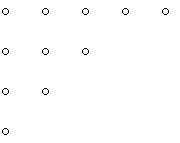A representation of the partition of 11 ($\{5,3,2,1\}$) using rows of dots

While these diagrams show partitions of 10 and 11 by reading across the rows, one also sees that these diagrams display partitions of 10, and 11, namely, 3,2,2,1 and 4,3,2,1,1 respectively, by reading in the vertical direction rather than the horizontal direction. Thus, each Ferrers's diagram gives rise to two partitions, which are called conjugate partitions. Some diagrams will read the same in both the horizontal and vertical directions; such partitions are called self-conjugate. Experiment to see if you can convince yourself that the number of self-conjugate partitions of $n$ is the same as the number of partitions of $n$ with odd parts that are all different!

The next figure collects Ferrers's diagrams for the partitions of small integers.Ferrers's diagrams of partitions of the integers starting with 1, lower right, and increasing to partitions of 7
Courtesy of Wikipedia

Often to get insights into mathematical phenomena one needs data. Here for example, complementing the previous figure, is a table of the ways to write the number $n$ as the sum of $k$ parts. For example, 8 can be written as a sum of two parts in 4 ways. These are the partitions of 8 which have two parts: $7+1$, $6+2$, $5+3$, and $4+4$.

 $n$ into $k$ parts 1 2 3 4 5 6 7 8 1 1 2 1 1 3 1 1 1 4 1 2 1 1 5 1 2 2 1 1 6 1 3 3 2 1 1 7 1 3 4 3 2 1 1 8 1 4 5 5 3 2 1 1 Fill in next row!!

Table: Partition of the number $n$ into $k$ parts

While many people have contributed to the development of the theory of partitions, the prolific Leonhard Euler (1707-1783) was one of the first.Leonhard Euler
Courtesy of Wikipedia

Euler was one of the most profound contributors to mathematics over a wide range of domains, including number theory, to which ideas related to partitions in part belongs. Euler showed a surprising result related to what today are called figurate numbers. In particular he discovered a result related to pentagonal numbers. Euler was fond of using power series (a generalized polynomial with infinitely many terms) which in the area of mathematics dealing with counting problems, combinatorics, is related to generating functions. If one draws a square array of dots, one sees $1, 4, 9, 16, \dots$ dots in the pattern that one draws. What happens when one draws triangular, pentagonal, or hexagonal arrays of dots? In the next two figures, we see a sample of the many ways one can visualize the pentagonal numbers: $1, 5, 12, 22, \dots$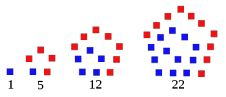(a)                                             (b)
Two ways of coding the pentagonal numbers
Courtesy of Wikipedia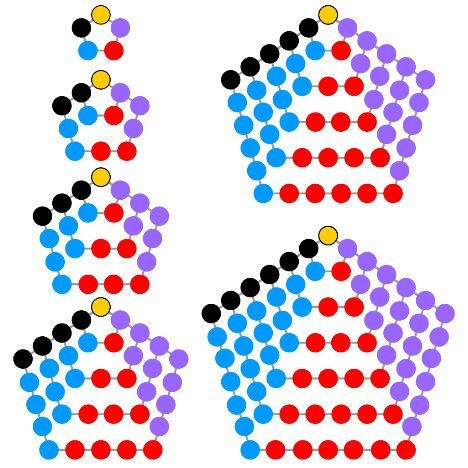The pentagonal numbers for side lengths of the pentagon from 2 to 6
Courtesy of Wikipedia

Godfrey Harold Hardy (1877-1947), Ramanujan and in more modern times, George Andrews and Richard Stanley have been important contributors to a deeper understanding of the patterns implicit in partitions and ways to prove that the patterns that are observed are universally correct.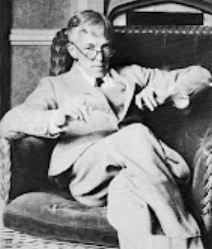Photo of G. H. Hardy
Courtesy of Wikipedia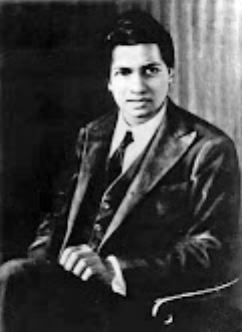Srinivasa Ramanujan
Courtesy of WikipediaPhoto of George Andrews
Courtesy of WikipediaPhoto of Richard Stanley
Courtesy of Wikipedia

What is worth noting here is that the methodology of mathematical investigations is both local and global. When one hits upon the idea of what can be learned by “decomposing’ something one understands in the hope of getting a deeper understanding, it also has implications in other environments where one uses the broader notion (decomposition). So understanding primes as building blocks encourages one to investigate primes locally in the narrow arena of integers but also makes one think about other kinds of decompositions that might apply to integers. We are interested in not only decompositions of integers from a multiplicative point of view but also decompositions of integers from an additive point of view. Here in a narrow sense one sees problems like the Goldbach Conjecture but in a broader sense it relates to the much larger playground of the partitions of integers. When one develops a new approach to looking at a situation (e.g. decomposing something into parts) mathematicians invariably try to "export" the ideas discovered in a narrow setting to something more global, including areas of mathematics that are far from where the original results were obtained. So if decomposition is useful in number theory, why not try to understand decompositions in geometry as well? Thus, there is a whole field of decompositions dealing with plane polygons, where the decompositions are usually called dissections.

As an example of a pattern which has been discovered relatively recently and which illustrates that there are intriguing mathematical ideas still to be discovered and explored, consider this table:

 Partition Distinct elements Number 1's 4 1 0 3+1 2 1 2+2 1 0 2+1+1 2 2 1+1+1+1 1 4 Total 7 7

See anything interesting—some pattern? Not all of the column entries are odd or even in the second and third columns. However, Richard Stanley noted that the sum of the second and third columns are equal! Both add to 7. And this is true for all values of $n$. How might one prove such a result? One approach would be to find a formula (function involving $n$) for the number of distinct elements in the partitions of $n$ and also find a formula for the number of 1's in the partitions of $n$. If these two formulas are the same for each value of $n$, then it follows that we have a proof of the general situation that is illustrated for the example $n = 4$ in the table above. However, it seems unlikely that there is a way to write down closed form formulas for either of these two different quantities. However, there is a clever approach to dealing with the observation above that is also related to the discovery of Georg Cantor (1845-1918) that there are sets with different sizes of infinity.

Consider the two sets, $\mathbb{Z}^+$ the set of all positive integers and the set $\mathbb{E}$ of all of the even positive integers. $\mathbb{Z}^+ = \{1,2,3,4,5, \dots\}$ and $\mathbb{E}=\{2,4,6,8,10,\dots\}$. Both of these are infinite sets. Now consider the table:

1 paired with 2
2 paired with 4
3 paired with 6
4 paired with 8
.
.
.

Note that each of the entries in $\mathbb{Z}^+$ will have an entry on the left in this "count" and each even number, the numbers in $\mathbb{E}$, will have an entry on the right in this "count." This shows that there is a one to one and onto way to pair these two sets, even though $\mathbb{E}$ is a proper subset of $\mathbb{Z}^+$ in the sense that every element of $\mathbb{E}$ appears in $\mathbb{Z}^+$ and there are elements in $\mathbb{Z}^+$ that don't appear in $\mathbb{E}$. There seems to be a sense in which $\mathbb{E}$ and $\mathbb{Z}^+$ have the same "size." This strange property of being able to pair elements of a set with a proper subset of itself can only happen for an infinite collection of things. Cantor showed that in this sense of size, often referred to as the cardinality of a set, some pairs of sets which seemed very different in size had the same cardinality (size). Thus, the set of positive integers Cantor showed had the same cardinality as the set of positive rational numbers (numbers of the form $a/b$ where $a$ and $b$ are positive integers with no common factor for $a$ and $b$). Remarkably, he was also able to show that the set of positive integers had a different cardinality from the set of real numbers. To this day there are questions dating back to Cantor's attempt to understand the different sizes that infinite sets can have that are unresolved.

What many researchers are doing for old and new results about partitions is to show that counting two collections, each defined differently but for which one gets the same counts, the equality of the counts can be shown by constructing a bijection between the two different collections. When such a one-to-one and onto correspondence (function) can be shown for any value of a positive integer n, then the two collections of things must have the same size. Such bijective proofs often show more clearly the connection between seemingly unrelated things rather than showing that the two different concepts can be counted with the same formula. These bijective proofs often help generate new concepts and conjectures.

Try investigating ways that decompositions might give one new insights into ideas you find intriguing.

### References

Those who can access JSTOR can find some of the papers mentioned above there. For those with access, the American Mathematical Society's MathSciNet can be used to get additional bibliographic information and reviews of some of these materials. Some of the items above can be found via the ACM Digital Library, which also provides bibliographic services.

Andrews, G.E., 1998. The theory of partitions (No. 2). Cambridge University Press.

Andrews, G.E. and Eriksson, K., 2004. Integer partitions. Cambridge University Press.

Atallah, Mikhail J., and Marina Blanton, eds. Algorithms and theory of computation handbook, volume 2: special topics and techniques. CRC Press, 2009.

Bóna, M. ed., 2015. Handbook of enumerative combinatorics (Vol. 87). CRC Press.

Fulton, Mr William, and William Fulton. Young tableaux: with applications to representation theory and geometry. No. 35. Cambridge University Press, 1997.

Graham, Ronald L. Handbook of combinatorics. Elsevier, 1995.

Gupta H. Partitions – a survey. Journal of Res. of Nat. Bur. Standards-B Math. Sciences B. 1970 Jan 1;74:1-29.

Lovász, László, József Pelikán, and Katalin Vesztergombi. Discrete mathematics: elementary and beyond. Springer Science & Business Media, 2003.

Martin, George E. Counting: The art of enumerative combinatorics. Springer Science & Business Media, 2001.

Matousek, Jiri. Lectures on discrete geometry. Vol. 212. Springer Science & Business Media, 2013.

Menezes, Alfred J., Paul C. Van Oorschot, and Scott A. Vanstone. Handbook of applied cryptography. CRC Press, 2018.

Pak, Igor. "Partition bijections, a survey." The Ramanujan Journal 12.1 (2006): 5-75.

Rosen, Kenneth H., ed. Handbook of discrete and combinatorial mathematics. CRC Press, 2017.

Rosen, Kenneth H., and Kamala Krithivasan. Discrete mathematics and its applications: with combinatorics and graph theory. Tata McGraw-Hill Education, 2012.

Sjöstrand, Jonas. Enumerative combinatorics related to partition shapes. Diss. KTH, 2007.

Stanley, Richard P. Ordered structures and partitions. Vol. 119. American Mathematical Soc., 1972.

Stanley, Richard P. "What is enumerative combinatorics?." Enumerative combinatorics. Springer, Boston, MA, 1986. 1-63.

Stanley, Richard P. "Enumerative Combinatorics Volume 1 second edition." Cambridge studies in advanced mathematics (2011).

Stanley, Richard P., and S. Fomin. "Enumerative combinatorics. Vol. 2, volume 62 of." Cambridge Studies in Advanced Mathematics (1999).

Stanley, Richard P. Catalan numbers. Cambridge University Press, 2015.

Toth, Csaba D., Joseph O'Rourke, and Jacob E. Goodman, eds. Handbook of discrete and computational geometry. CRC Press, 2017.